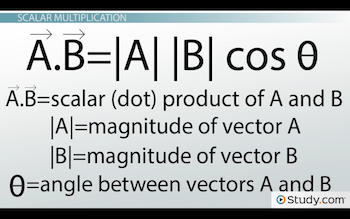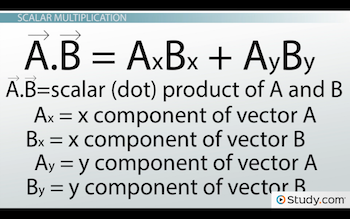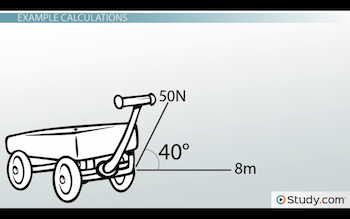# Scalar Multiplication of Vectors: Definition & Calculations

An error occurred trying to load this video.

Try refreshing the page, or contact customer support.

Coming up next: Vector Subtraction (Geometric): Formula & Examples

### You're on a roll. Keep up the good work!

Replay
Your next lesson will play in 10 seconds
• 0:04 When Do We Multiply Vectors?
• 1:20 Scalar Multiplication
• 3:15 Example Calculations
• 4:56 Lesson Summary

Want to watch this again later?

Timeline
Autoplay
Autoplay
Speed

#### Recommended Lessons and Courses for You

Lesson Transcript
Instructor: David Wood

David has taught Honors Physics, AP Physics, IB Physics and general science courses. He has a Masters in Education, and a Bachelors in Physics.

After watching this video, you will be able to explain what scalar multiplication is and complete basic calculations involving the scalar multiplication of vectors. A short quiz will follow.

## When Do We Multiply Vectors?

Some numbers in physics have a direction and some don't. You can be cycling down a trail at 3 miles per hour north, but the thermometer you happen to have on your bike (because you love science so much) can't experience a temperature of 32 degrees north. Temperature has no direction. Because of that, we call it a scalar quantity, or a quantity that has magnitude (size), but no direction. The opposite of this is a vector, like velocity, or force, or magnetic field, which is a quantity that has both a magnitude (size) and direction.

There are hundreds of equations in physics, and they contain a mixture of scalars and vectors. Whenever two vectors are multiplied together in one of these equations, like force multiplied by displacement (which is work), or the velocity of a charge multiplied by magnetic field (which is related to the magnetic force), we can multiply them in two different ways: vector multiplication (otherwise known as a cross product) and scalar multiplication (otherwise known as a dot product).

Which one do you do? That depends on the exact situation. Some equations call for a dot product, while others call for a cross product. Generally, if the answer you're looking for is a scalar quantity, it will be a scalar product. But, if the answer you're looking for is a vector quantity, it will be a vector product.

## Scalar Multiplication

Work is probably the simplest example of a scalar multiplication of vectors. Work is equal to displacement multiplied by force, or in other words, how far an object moves multiplied by the force applied to make it move. Both displacement and force are vectors.

But, if the force was applied at an angle... say, by pushing diagonally down on a broom as it skirts across the floor, we can make the definition of work more specific. We might say that work is equal to the displacement multiplied by the component of the force that acts in the direction of motion. If you're pushing the broom down at an angle, then it's only the part of the force that points along the floor that we're interested in. Whenever that is the case in a physics situation, we're doing scalar multiplication; we're completing a dot product. So in summary, a scalar multiplication is where you multiply one vector by the component of a second vector that acts in the direction of the first vector.

There are two main equations for calculating a dot product. If you have the overall magnitudes and angles of the vector, you use this equation:So, if you're multiplying vector A by vector B, you take the magnitude of vector A, multiply it by the magnitude of vector B, and multiply that by the cosine of the angle between them. So, this would be like taking your displacement and multiplying it by F cosine theta, the component of the force that acts in the direction of the displacement.

But, what if you're given a quantity in component form? Maybe you don't know the overall magnitude of a vector, but you do know the x and y components of A and the x and y components of B. In that case, you would use the equation below, and it'll work out exactly the same. Just multiply the two x components together and the two y components (and, if you were in 3 dimensions, you would do the same with the two z components), and add them all up.Aside from work, other examples of scalar products include magnetic potential energy (which is dipole moment multiplied by magnetic field), magnetic flux (magnetic field multiplied by area), and power (force multiplied by velocity).

## Example Calculations

Okay, now let's go through an example.

Let's say you're dragging your cousin along the street in a wagon. Because of how tall you are, you can't help but pull up at an angle. It would hurt your knees to bend down all the time. If you pull at an angle of 40 degrees to the horizontal with a force of 50 newtons, and the cart moves 8 meters in the positive x-direction, how much work is done in Joules?To unlock this lesson you must be a Study.com Member.

### Register to view this lesson

Are you a student or a teacher?

### Unlock Your Education

#### See for yourself why 30 million people use Study.com

##### Become a Study.com member and start learning now.
Back
What teachers are saying about Study.com

### Earning College Credit

Did you know… We have over 160 college courses that prepare you to earn credit by exam that is accepted by over 1,500 colleges and universities. You can test out of the first two years of college and save thousands off your degree. Anyone can earn credit-by-exam regardless of age or education level.